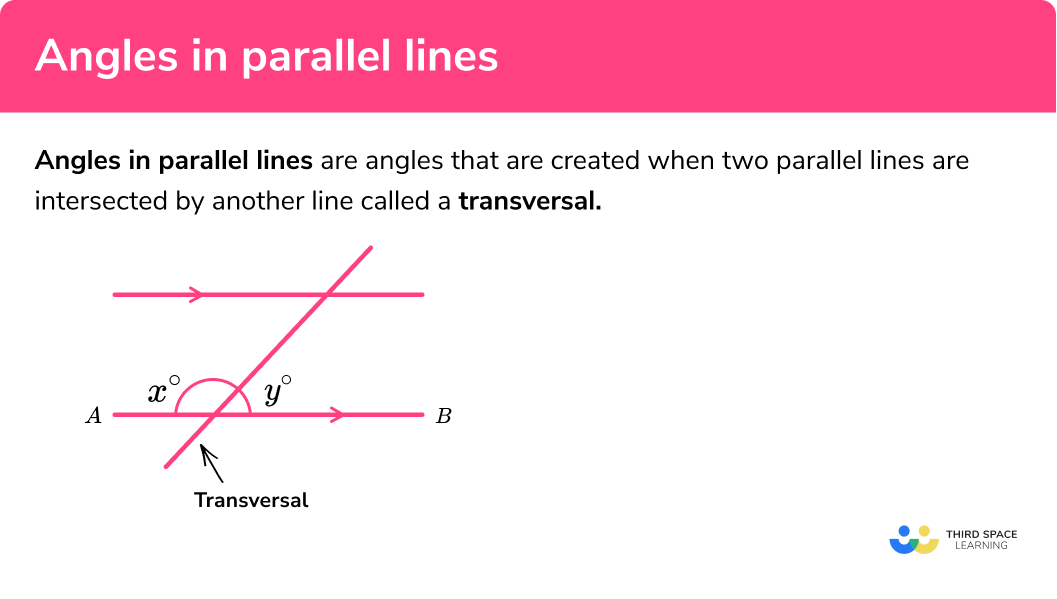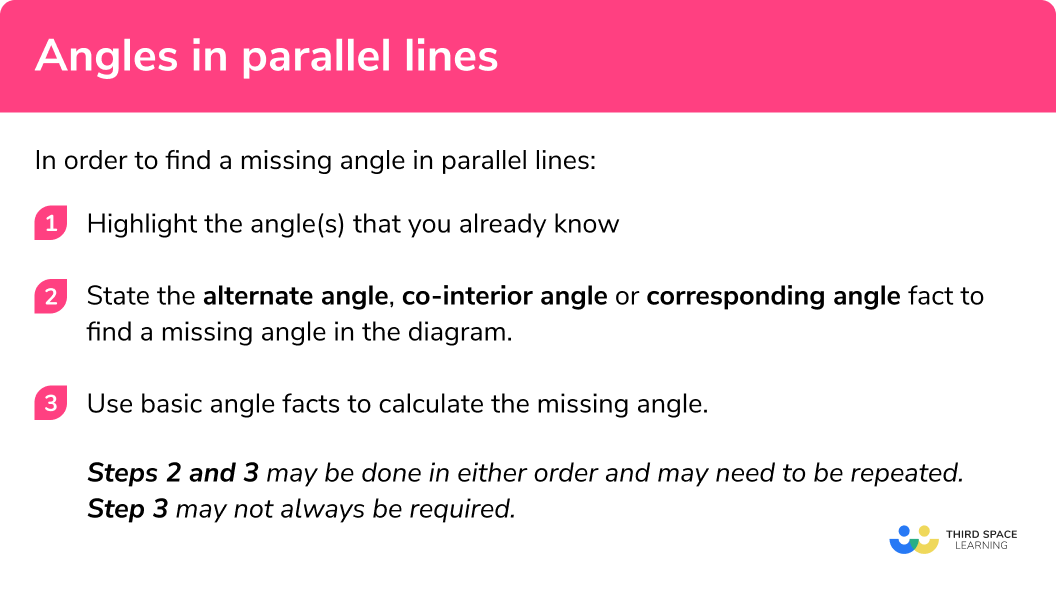GCSE Maths Geometry and Measure Angles

Angles In Parallel Lines

# Angles In Parallel Lines

Here we will learn about angles in parallel lines including how to recognise angles in parallel lines, use angle facts to find missing angles in parallel lines, and apply angles in parallel lines facts to solve algebraic problems.

There are also angles in parallel lines worksheets based on Edexcel, AQA and OCR exam questions, along with further guidance on where to go next if you’re still stuck.

## What are angles in parallel lines?

Angles in parallel lines are angles that are created when two parallel lines are intersected by another line called a transversal.

We can use the information given in the diagram to find any angle around the intersecting transversal.

To do this, we use three facts about angles in parallel lines:

Alternate angles, co-Interior angles, and corresponding angles.

Properties of parallel lines

• Alternate angles are equal:

Sometimes called ‘Z angles’.

• Corresponding angles are equal:

Sometimes called ‘F angles’

• Co-interior angles add up to 180^o:

Sometimes called ‘C angles’.

### What are angles in parallel lines?### Key angle facts

To explore angles in parallel lines we will need to use some key angle facts.

Angles on a straight linex+y=180^o

(The sum of angles on a straight line equals 180^o )

Angles around a pointe+f+g+h=360^o

(The sum of angles around a point
equals 360^o )

Angles in a triangleA+B+C=180^o

(The sum of angles in a triangle equals 180^o )

Vertically Opposite angles(Vertically opposite angles are the same
size)

### Angles in parallel lines

We know that vertically opposite angles are equal and we can show this around a point within our parallel lines:

If we extend the transversal line so that it crosses more parallel lines, the angles that are made are maintained throughout the diagram for any line that is parallel to the original line AB.

Top Tip: for the same intersecting transversal, all the acute angles are the same size, and all the obtuse angles are the same size.

We group these angles into three separate types called alternate angles, co-interior angles and corresponding angles.

### Alternate angles

Alternate angles are angles that occur on opposite sides of the transversal line and have the same size.

Each pair of alternate angles around the transversal are equal to each other.
The two angles can either be alternate interior angles or alternate exterior angles.

Other examples of alternate angles:We can often spot interior alternate angles by drawing a Z shape:

Step-by-step guide: Alternate angles

### Corresponding angles

The pairs of angles formed on the same side of the transversal that are either both obtuse or both acute and are called corresponding angles and are equal in size.

Each pair of corresponding angles on the same side of the intersecting transversal are equal to each other.

Other examples of corresponding angles:We can often spot interior corresponding angles by drawing an F shape:

Step-by-step guide: Corresponding angles

### Co-Interior angles

Co-interior angles on the same side of an intersecting transversal add to 180^o .

g+h= 180^o

Other examples of co-interior angles:i+j=180ok+l=180om+n=180o

We can often spot interior co-interior angles by drawing a C shape.

Step-by-step guide: Co-interior angles

## How to find a missing angle in parallel lines

In order to find a missing angle in parallel lines:

1 Highlight the angle(s) that you already know.

2 State the alternate angle, co-interior angle or corresponding angle fact to find a missing angle in the diagram.

3 Use basic angle facts to calculate the missing angle.

Steps 2 and 3 may be done in either order and may need to be repeated.
Step 3 may not always be required.

### How to find a missing angle in parallel lines## Angles in parallel lines examples

For each stage of the calculation we must clearly state any angle facts that we use.

### Example 1: alternate angles

Calculate the size of the missing angle \theta . Justify your answer.

1. Highlight the angle(s) that you already know.

2State the alternate angle, co-interior angle or corresponding angle fact to find a missing angle in the diagram.

Here we can label the alternate angle on the diagram as 50^o .

3Use a basic angle fact to calculate the missing angle.

Here as \theta is on a straight line with 50^o ,

\theta =180^o-50^o

\theta =130^o

### Example 2: co-Interior angles

Calculate the size of the missing angle \theta . Justify your answer.

Here we can label the co-interior angle on the diagram as 60^o as 120+60= 180^o .

We can see that as \theta is vertically opposite to 60^o ,

\theta =60^o .

### Example 3: corresponding angles

Calculate the size of the missing angle \theta . Justify your answer.

Here we can label the corresponding angle on the diagram as 75^o .

Here as \theta is on a straight line with 75^o ,

\theta =180-75

\theta =105^o .

### Example 4: multiple-steps

Calculate the size of the missing angle \theta . Show all your working.

Opposite angles are equal so we can label the angle 110^o .

Co-interior angles add up to 180^o . Here 180-110=70^o .

θ is corresponding to 70+35 so θ = 70+35 = 105^o .

### Example 5: similar triangles

Show that the two triangles are similar.Here, we can see that the two angles highlighted in green are on a straight line and so their sum is 180^o . This gives us the missing angle of 70^o .

We can also see there is a vertically opposite angle at the centre of the diagram. This is also 90^o .

The smaller triangle now has a missing angle of 20^o as angles in a triangle add to equal 180^o .

By stating the alternate angles from 70^o and 20^o we can see that θ=20^o and the other angle in the triangle is 70^o . The two triangles contain the same angles and are therefore similar.

### Example 6: angles in parallel lines including algebra

Given that the sum of angles on a straight line is equal to 180^o , calculate the value of x . Hence or otherwise, calculate the size of angle 4x+30 .

Here we can state that 20^o is corresponding to the original angle.

As the sum of angles on a straight line is 180^o, we have:.

4x+30+20=180^o

4x=130

x=32.5^o

Now that x=32.5^o,

4x+30=4(32.5)+30

=160^o

### Common misconceptions

• Mixing up angle facts

There are a lot of angle facts and it is easy to mistake alternate angles with corresponding angles. To prevent this from occurring, think about the alternate angles being on the alternate sides of the line.

• Using a protractor to measure an angle.

Most diagrams are not to scale and so using a protractor will not result in a correct answer unless it is a coincidence.

### Practice angles in parallel lines questions

1. Calculate the size of angle \theta\theta = 100^{\circ}\theta =80^{\circ}\theta =138^{\circ}\theta =42^{\circ}Using corresponding angles, we can see the angle 42^{\circ}:We can then use angles on a straight line:

\theta=180-42= 138^{\circ}

2. Calculate the size of angle \thetaNot to scale

\theta = 62^{\circ}\theta =118^{\circ}\theta =298^{\circ}\theta =28^{\circ}Using co-interior angles, we can calculate:

180-62=118^{\circ}Then we can label the corresponding angle

118^{\circ}:Since opposite angles are equal,

\theta=118^{\circ}

3. Calculate the angle  \thetaNot to scale

\theta =21^{\circ}\theta =159^{\circ}\theta =69^{\circ}\theta =79.5^{\circ}Using opposite angles, we can see the angle 21^{\circ}.

Next we can label the alternate angle 21^{\circ}:We can then use the fact that it is an isosceles triangle and so two base angles are equal:

\theta=\frac{180-21}{2}=79.5^{\circ}

4. Calculate the size of angle \thetaNot to scale

\theta =23^{\circ}\theta =67^{\circ}\theta =113^{\circ}\theta =157^{\circ}Using angles on a straight line, we can calculate:

180-(90+67)=23^{\circ}We can then use alternate angles to see that

\theta=23^{\circ}

5. Calculate the size of angle \thetaNot to scale

\theta =151^{\circ}\theta =88^{\circ}\theta =121^{\circ}\theta =59^{\circ}Using angles on a straight line we can calculate the angles  92^{\circ} and 59^{\circ}:Then the other angle in the triangle is:

180-(92+59)=29^{\circ}.

Using angles on a straight line we can calculate:

180-29=151^{\circ}.Finally, using corresponding angles, we can see that:

\theta=151^{\circ}.

6. By calculating the value of x , find the value of \thetaNot to scale

\theta =150^{\circ}\theta =90^{\circ}\theta =30^{\circ}\theta =85^{\circ}30x-25 and 20x+5 are alternate angles. Therefore, we can write:

30x-25=20x+5.

We can then solve this to find x :

\begin{aligned} 30x-25&=20x+5\\ 10x-25&=5\\ 10x&=30\\ x&=3 \end{aligned}

Given that x=3 ,

30 \times 3-25=65

Using opposite angles, we can see that the angle inside the triangle is 65^{\circ}:Using angles in a triangle, we can calculate the third angle in the triangle:

180-(65+30)=85^{\circ}.

Then using opposite angles,

\theta=85^{\circ}

### Angles in parallel lines GCSE questions

1. (a) Below is a diagram showing two parallel lines intersected by a transversal:Write an equation connecting r and s.

(b) Given that the ratio of the angles r : s is equivalent to 3 : 5 , write another equation connecting r and s.

(2 marks)

(a)  r + s = 180

(1)

(b)  5r = 3s

(1)

2. Lines AB and CD are parallel.(a) By finding the value of x , calculate the exact value of z^{\circ} .

(b) Calculate the value of y^{\circ}.

(4 marks)

(a)

5x – 10 = 4x – 2

(1)

x = 8^{\circ}

(1)

4 × 8  −  2 = z = 30^{\circ}

(1)

(b)

y = 180 – 30 = 150^{\circ}

(1)

## Learning checklist

You have now learned how to:

• apply the properties of angles at a point, angles at a point on a straight line, vertically opposite angles
• understand and use the relationship between parallel lines and alternate and corresponding angles

## Still stuck?

Prepare your KS4 students for maths GCSEs success with Third Space Learning. Weekly online one to one GCSE maths revision lessons delivered by expert maths tutors.

Find out more about our GCSE maths tuition programme.